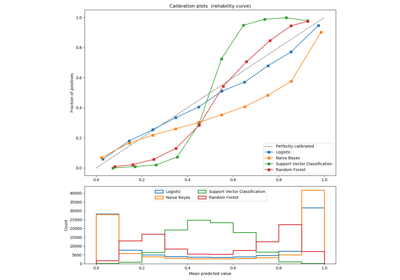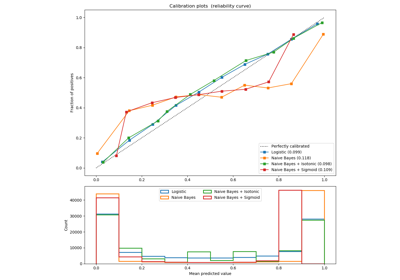# sklearn.calibration.calibration_curve¶

sklearn.calibration.calibration_curve(y_true, y_prob, normalize=False, n_bins=5)[source]

Compute true and predicted probabilities for a calibration curve.

The method assumes the inputs come from a binary classifier.

Calibration curves may also be referred to as reliability diagrams.

Read more in the User Guide.

Parameters: y_true : array, shape (n_samples,) True targets. y_prob : array, shape (n_samples,) Probabilities of the positive class. normalize : bool, optional, default=False Whether y_prob needs to be normalized into the bin [0, 1], i.e. is not a proper probability. If True, the smallest value in y_prob is mapped onto 0 and the largest one onto 1. n_bins : int Number of bins. A bigger number requires more data. Bins with no data points (i.e. without corresponding values in y_prob) will not be returned, thus there may be fewer than n_bins in the return value. prob_true : array, shape (n_bins,) or smaller The true probability in each bin (fraction of positives). prob_pred : array, shape (n_bins,) or smaller The mean predicted probability in each bin.

References

Alexandru Niculescu-Mizil and Rich Caruana (2005) Predicting Good Probabilities With Supervised Learning, in Proceedings of the 22nd International Conference on Machine Learning (ICML). See section 4 (Qualitative Analysis of Predictions).

## Examples using sklearn.calibration.calibration_curve¶Comparison of Calibration of ClassifiersProbability Calibration curves